SSC  >  Test: General Intelligence & Reasoning- 2

# Test: General Intelligence & Reasoning- 2

Test Description

## 50 Questions MCQ Test Mock Test Series of SSC JE Civil Engineering | Test: General Intelligence & Reasoning- 2

Test: General Intelligence & Reasoning- 2 for SSC 2023 is part of Mock Test Series of SSC JE Civil Engineering preparation. The Test: General Intelligence & Reasoning- 2 questions and answers have been prepared according to the SSC exam syllabus.The Test: General Intelligence & Reasoning- 2 MCQs are made for SSC 2023 Exam. Find important definitions, questions, notes, meanings, examples, exercises, MCQs and online tests for Test: General Intelligence & Reasoning- 2 below.
Solutions of Test: General Intelligence & Reasoning- 2 questions in English are available as part of our Mock Test Series of SSC JE Civil Engineering for SSC & Test: General Intelligence & Reasoning- 2 solutions in Hindi for Mock Test Series of SSC JE Civil Engineering course. Download more important topics, notes, lectures and mock test series for SSC Exam by signing up for free. Attempt Test: General Intelligence & Reasoning- 2 | 50 questions in 50 minutes | Mock test for SSC preparation | Free important questions MCQ to study Mock Test Series of SSC JE Civil Engineering for SSC Exam | Download free PDF with solutions
 1 Crore+ students have signed up on EduRev. Have you?
Test: General Intelligence & Reasoning- 2 - Question 1

### In the following question, select the related word from the given alternatives. College : Dean ∷ Museum : ?

Detailed Solution for Test: General Intelligence & Reasoning- 2 - Question 1

Dean is the in charge of the college.

Similarly, Curator is the in charge of the museum.

Test: General Intelligence & Reasoning- 2 - Question 2

### Select the related word from the alternatives: Pig : Sty :: Dog : ?

Detailed Solution for Test: General Intelligence & Reasoning- 2 - Question 2

Pig is an animal which lives in a sty. Dog lives in a Kennel,

Hence the answer is option Kennel.

Test: General Intelligence & Reasoning- 2 - Question 3

### In the following question, select the related word pair from the given alternatives. Football : Outdoor : : ? : ?

Detailed Solution for Test: General Intelligence & Reasoning- 2 - Question 3

‘Football’ is an ‘Outdoor’ game. Similarly, ‘Snooker’ is an ‘Indoor’ game.

Test: General Intelligence & Reasoning- 2 - Question 4

Select the related number from the given alternatives.

5 : -10 : : -8 : ?

Detailed Solution for Test: General Intelligence & Reasoning- 2 - Question 4

The pattern followed is: x : -2x

→ 5 : -2 × 5 = -10

Similarly,

→ -8 : -2 × (-8) = 16

Hence, the correct answer is 16

Test: General Intelligence & Reasoning- 2 - Question 5

Directions: In each of the following questions, select the related number from the given alternatives.

5 : 24 : : 23 : ?

Detailed Solution for Test: General Intelligence & Reasoning- 2 - Question 5

In 5 : 24
→ 5 × 5 = 25 – 1 = 24
Similarly;
→ 23 × 5 = 115 – 1 = 114
Hence, 5 : 24 : : 23 : 114

Test: General Intelligence & Reasoning- 2 - Question 6

In the following question, select the related numbers from the given alternatives.

J : 90 ∷ N : ?

Detailed Solution for Test: General Intelligence & Reasoning- 2 - Question 6

With reference to English alphabets,

J = 10

J2 – J = 102 – 10 = 100 – 10 = 90.

Similarly,

N = 14

N2 – N = 142 – 14 = 196 – 14 = 182.

Test: General Intelligence & Reasoning- 2 - Question 7

Select the related letters from the given alternatives.

ZWU : TQO : : LIG : ?

Detailed Solution for Test: General Intelligence & Reasoning- 2 - Question 7

In ZWU : TQO

Z – 3 = W; W – 2 = U

U – 1 = T

T – 3 = Q; Q – 2 = O

Similarly, in LIG : ?

L – 3 = I; I – 2 = G

G – 1 = F

F – 3 = C; C – 2 = A

Test: General Intelligence & Reasoning- 2 - Question 8

Directions: In the following question, select the odd letters from the given alternatives.

ACEG : DFHJ :: QSUW : ?

Test: General Intelligence & Reasoning- 2 - Question 9

Select the related letters from the given alternatives.

VTHB : KJDA ∷ ? : CMEI

Detailed Solution for Test: General Intelligence & Reasoning- 2 - Question 9

We know that position of alphabet is A = 1; B = 2; C = 3…and so on.

In VTHB : KJDA

VTHB their position in English alphabet. ⇒ V = 22, T = 20, H = 8, B = 2

KJDA their position in English alphabet. ⇒ K = 11, J = 10, D = 4, A = 1

Similarly,

FZJR their position in English alphabet ⇒ F = 6, Z = 26, J = 10, R = 18

CMEItheir position in English alphabet. ⇒ C = 3, M = 13, E = 5, I = 9

Hence, “FZJR” is missing term.

Test: General Intelligence & Reasoning- 2 - Question 10

In the following question, select the odd number from the given alternatives.

Detailed Solution for Test: General Intelligence & Reasoning- 2 - Question 10

The logic followed is:

Sum of all the digits in the number is not a prime number, except in option 1.

1) 788 → 7 + 8 + 8 = 23, which is a prime number.

2) 654 → 6 + 5 + 4 = 15, which is not a prime number.

3) 984 → 9 + 8 + 4 = 21, which is not a prime number.

4) 824 → 8 + 2 + 4 = 14, which is not a prime number.

Hence, “788” is the odd one among the given alternatives.

Test: General Intelligence & Reasoning- 2 - Question 11

Find out the odd number from the given alternatives.

Detailed Solution for Test: General Intelligence & Reasoning- 2 - Question 11

The logic followed is:

1) 29 → 52 + 4

2) 85 → 92 + 4

3) 147 → 122 + 3

4) 125 → 112 + 4

Except “147” all other numbers are in the form n2 + 4.

Hence, “147” is the odd one among the given alternatives.

Test: General Intelligence & Reasoning- 2 - Question 12

Directions: Find the odd number pair from the given alternatives.

Detailed Solution for Test: General Intelligence & Reasoning- 2 - Question 12

The two digits of the given number are reversed and its pair is written with the reversed digits.

So 46 - 54 has to be 46 - 64.

Hence pair 46 - 54 is odd one.

Test: General Intelligence & Reasoning- 2 - Question 13

In the following question, select the odd word pair from the given alternatives.

Detailed Solution for Test: General Intelligence & Reasoning- 2 - Question 13

All are there part of colour except colour - green.

Test: General Intelligence & Reasoning- 2 - Question 14

Directions: Choose the word which is different from the rest.

Detailed Solution for Test: General Intelligence & Reasoning- 2 - Question 14

All except Bear belong to the cat family.

Hence, Bear is different from rest.

Test: General Intelligence & Reasoning- 2 - Question 15

Directions: In question find the word which is different from the given alternatives.

Detailed Solution for Test: General Intelligence & Reasoning- 2 - Question 15

→ Keyboard, Mouse and Monitor are hardware component of computer but MySql is software to store data in database.

Test: General Intelligence & Reasoning- 2 - Question 16

In the following question, select the odd letter/letters from the given alternatives.

Detailed Solution for Test: General Intelligence & Reasoning- 2 - Question 16

The pattern followed here is,

1) T – 3 = Q, Q – 3 = N, N – 3 = K

2) U – 3 = R, R – 3 = O (N), O (N) – 3 = L (M)

3) V – 3 = S, S – 3 = P, P – 3 = M

4) L – 3 = I, I – 3 = F, F – 3 = C

Hence, the odd one is ‘URNM’.

Test: General Intelligence & Reasoning- 2 - Question 17

In the following question, select the odd letters from the given alternatives.

Detailed Solution for Test: General Intelligence & Reasoning- 2 - Question 17

1) M + 7 = T;

2) C + 7 = J;

3) S + 7 = Z;

4) F + 8 = N

Hence, FN is odd one out.

Test: General Intelligence & Reasoning- 2 - Question 18

In the following question, select the odd word pair from the given alternatives.

Detailed Solution for Test: General Intelligence & Reasoning- 2 - Question 18

In all the pairs, the 1st word is an Antonym of 2nd word i.e.

Up - Down → Up is an antonym of Down.

Increase - Decrease → Increase is an antonym of Decrease.

Cry - Laugh → Cry is an antonym of Laugh.

But in, Walking - Running → Walking is not an antonym of Running.

Thus, Walking - Running is the odd pair.

Test: General Intelligence & Reasoning- 2 - Question 19

A series is given, with one term missing. Choose the correct alternative from the given ones that will complete the series.

8, 10, 30, 32, 52, 54, 74, 76, 96, ?

Detailed Solution for Test: General Intelligence & Reasoning- 2 - Question 19

The given series is,

8 + (2) → 10 + (20) → 30 + (2) → 32 + (20) → 52 + (2) → 54 + (20) → 74 + (2) → 76 + (20) → 96 + (2) → 98

Test: General Intelligence & Reasoning- 2 - Question 20

Direction: Complete the following number series.

1, 28, 92, 217, 433, ?

Detailed Solution for Test: General Intelligence & Reasoning- 2 - Question 20

1 + 33 = 28,

28 + 43 = 92,

92 + 53 = 217,

217+ 63 = 433,

433 + 73 = 776

Hence the question mark is replaced by 776.

Test: General Intelligence & Reasoning- 2 - Question 21

Directions: In the following question, choose the correct alternative from the given ones that will complete the series:

21, 24, 32, 45, 63, ?

Test: General Intelligence & Reasoning- 2 - Question 22

A series is given with one term missing. Select the correct alternative from the given ones that will complete the series.

LMK, FGE, ZAY, TUS, ?

Detailed Solution for Test: General Intelligence & Reasoning- 2 - Question 22

The pattern followed here is,

L - 6 = F; F - 6 = Z; Z - 6 = T; T - 6 = N

M - 6 = G; G - 6 = A; A - 6 = U; U - 6 = O

K - 6 = E; E - 6 = Y; Y - 6 = S; S - 6 = M

Hence, NOM is the correct answer.

Test: General Intelligence & Reasoning- 2 - Question 23

Which of the following terms follows the trend of the given list?

aAAAAAA, AaAAAAA, AAaAAAA, AAAaAAA, AAAAaAA, _________.

Detailed Solution for Test: General Intelligence & Reasoning- 2 - Question 23

According to the sequence, ‘a’ is shifting one position ahead of its next position at every step. Thus next term would be ‘AAAAAaA’.

Test: General Intelligence & Reasoning- 2 - Question 24

Direction: Complete the series.

AZBC, BYCD, CXDE, ?

Test: General Intelligence & Reasoning- 2 - Question 25

If PROMISE is coded as OPRSIME, then FRIENDS is coded as

Test: General Intelligence & Reasoning- 2 - Question 26

In a certain code language,

'mxy das zci' means 'good little frock'

'Jmx cos zci' means 'girl behaves good'

'das ajp cos' means 'little girl fell'

Which word in that language stands for 'frock'?

Test: General Intelligence & Reasoning- 2 - Question 27

Rasik walked 20 m towards north. Then he turned right and walks 30 m. Then he turns right and walks 35 m. Then he turns left and walks 15 m. Finally he turns left and walks 15 m. In which direction and how many metres is he from the starting position?

Test: General Intelligence & Reasoning- 2 - Question 28

Richa is standing North of Amar and East of Tia and Soha is east of Amar and North - East of Monu and Sonu is South Tia and South- west of Amar. Monu is in which direction from Tia ?

Test: General Intelligence & Reasoning- 2 - Question 29

Directions: Select the missing number from the given responses.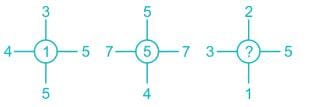Detailed Solution for Test: General Intelligence & Reasoning- 2 - Question 29

The central number is found by first finding the sum of numbers opposite to each other and then finding the difference between the resulting sums.

In the first case it is (4 + 5) – (3 + 5) = 1

Similarly, (7 + 7) – (5 + 4) = 5

So, (3 + 5) – (1 + 2) = 5

Hence the missing number is 5.

Test: General Intelligence & Reasoning- 2 - Question 30

Which of the following words will come third if arranged according to the English dictionary?

Detailed Solution for Test: General Intelligence & Reasoning- 2 - Question 30

The correct order will be:

Prawn → Prayer → Prick → Pride

Thus the third word is Prick.

Test: General Intelligence & Reasoning- 2 - Question 31

A is 2 years older to B. B is 5 years younger to C. C is 3 years older to D. D is 6 years younger to E. Who is the eldest?

Detailed Solution for Test: General Intelligence & Reasoning- 2 - Question 31

According to the question the relation between the ages of A, B, C, D and E are

A = B + 2

B = C - 5

C = D + 3

D = E - 6

Let the age of E be 10 years

D = 10 - 6 = 4 years

C = D + 3 = 4 + 3 = 7 years

B = C - 5 = 7 - 5 = 2 years

A = B + 2 = 2 + 2 = 4 years

Hence, E is the eldest.

Test: General Intelligence & Reasoning- 2 - Question 32

Select the set of symbols which can be fitted correctly in the equation.

8___4___2___6___3 = 32

Detailed Solution for Test: General Intelligence & Reasoning- 2 - Question 32

1) ×, –, + , ÷ ⇒ 8 × 4 – 2 + 6 ÷ 3 = 32 – 2 + 2 = 32 (True)

2) + , ×, ÷, – ⇒ 8 + 4 × 2 ÷ 6 – 3 = 8 – 4/3 – 3 ≠ 32 (False)

3) + , + , ×, – ⇒ 8 + 4 + 2 × 6 – 3 = 12 + 12 – 2 ≠ 32 (False)

4) –, x, ÷, + ⇒ 8 – 4 × 2 + 6 ÷ 3 = 8 – 8 + 2 ≠ 32 (False)

Hence, “×, –, + , ÷” give correct equation.

Test: General Intelligence & Reasoning- 2 - Question 33

If "–" denotes "divided by", "+" denotes "subtracted from", "×" denotes "added to" and "÷" denotes "multiplied by", then 4 ÷ 16 × 5 + 4 – 2 = ?

Detailed Solution for Test: General Intelligence & Reasoning- 2 - Question 33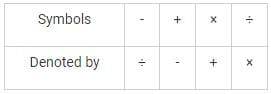Given expression: 4 ÷ 16 × 5 + 4 – 2

After changing: 4 × 16 + 5 - 4 ÷ 2

= 64 + 5 – 2

= 67

Hence, 67 is the correct answer.

Test: General Intelligence & Reasoning- 2 - Question 34

F and G are brothers. P and Q are sisters. F’s son is Q’s brother. How G related to P?

Test: General Intelligence & Reasoning- 2 - Question 35

Arrange the following words in the sequence in which they occur in dictionary:

1. Brook,

2. Bandit,

3. Boisterous,

4. Baffle,

5. Bright

Detailed Solution for Test: General Intelligence & Reasoning- 2 - Question 35

Clearly, the correct alphabetical order to the given words is baffle,

bandit, boisterous, bright, brook,

Thus, the correct sequence is 4, 2, 3, 5, 1.

Test: General Intelligence & Reasoning- 2 - Question 36

Arrange the words in the alphabetical order and tick the one that comes second

Detailed Solution for Test: General Intelligence & Reasoning- 2 - Question 36

The given words can be arrange in the alphabetical order as:

eager, ecstasy, emergency, and explosion

clearly, ecstasy comes second.

Test: General Intelligence & Reasoning- 2 - Question 37

Select the word which cannot be formed using the letters of the given word

REJUVENATION

Detailed Solution for Test: General Intelligence & Reasoning- 2 - Question 37

JUTE → can be formed → REJUVENATION

NATION → can be formed → REJUVENATION

REVISION → cannot be formed as there is no S and only one I

NATIVE → can be formed → EJUVENATION

Test: General Intelligence & Reasoning- 2 - Question 38

Which of the following venn diagrams correctly illustrates the relationship among the classes women,  Teacher,  Mother?

Test: General Intelligence & Reasoning- 2 - Question 39

Directions: Study the following information carefully and answer the given questions.

P # Q means P is the father of Q.

P \$ Q means P is the mother of Q.

P @ Q means P is the sister of Q.

P % Q means P is the brother of Q.

If ‘S @ T \$ U % V’ then S is V’s

Test: General Intelligence & Reasoning- 2 - Question 40

Find the part which represents those actors who are also singers.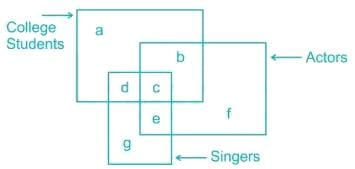Test: General Intelligence & Reasoning- 2 - Question 41

Consider the given statement/s to be true and decide which of the given conclusions/assumptions can definitely be drawn from the given statements.

Statements:

I. All elephants are men.

II. All men are socks.

Conclusions:

I. Some socks are elephants.

II. All elephants are socks.

Test: General Intelligence & Reasoning- 2 - Question 42

In the following question, three statements are given, followed by three conclusions. You have to consider the statements to be true even if they seem to be at variance from commonly known facts. You have to decide which of the given conclusions, if any, follow from the given statements.

Statement:

Some benches are tables

All chairs are stools

All tables are chairs

Some stools are tables

Conclusions:

I. Some benches are stools

II. Some benches are not chairs is a possibility.

III. All chairs are tables

IV. All tables are stools

Test: General Intelligence & Reasoning- 2 - Question 43

Among the four answer figures, which one can be formed from the cut out pieces gives below in.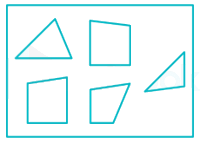Test: General Intelligence & Reasoning- 2 - Question 44

If a mirror is placed on the line MN, then which of the answer figures is the right image of the given figure?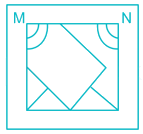Test: General Intelligence & Reasoning- 2 - Question 45

From the given answer figures, select the one in which the question figure is hidden/embedded.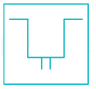Detailed Solution for Test: General Intelligence & Reasoning- 2 - Question 45

From the figure we can observe that the figure in the question is hidden in the option A.

In fig B no such pattern is there.

In fig C, the top left part of the pattern is missing.

In fig D, the top right part of the pattern is missing.

So, the correct option is A.

Test: General Intelligence & Reasoning- 2 - Question 46

Which answer figure will complete the pattern in the question figure?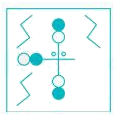Test: General Intelligence & Reasoning- 2 - Question 47

Which digit will appear on the face opposite to face with number 4?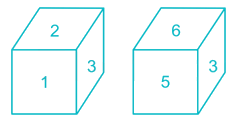Test: General Intelligence & Reasoning- 2 - Question 48

A piece of paper is folded and punched as shown below in the question figures. from the given answer figures, indicate how it will appear when opened.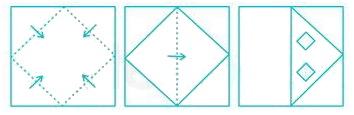Test: General Intelligence & Reasoning- 2 - Question 49

Directions: In the following question, find the missing number from the given responses.

6    9    8

4    6    6

2    3    4

12  ?    12

Detailed Solution for Test: General Intelligence & Reasoning- 2 - Question 49

The relation is as shown below

6 × 4 = 24 ÷ 2 = 12

8 × 6 = 48 ÷ 4 = 12

Similarly,

9 × 6 = 54 ÷ 3 = 18

Hence, the missing number is 18.

Test: General Intelligence & Reasoning- 2 - Question 50

A word is represented by only one set of numbers as given in any one of the alternatives. The sets of numbers given in the alternatives are represented by two classes of alphabets as shown in the given two matrices. The columns and rows of Matrix – I are numbered from 0 to 4 and that of Matrix – II are numbered from 5 to 9. A letter from these matrices can be represented first by its row and next by its column, for example, ‘A’ can be represented by 20, 43, etc. and ‘U’ can be represented by 68, 87, etc. Similarly, you have to identify the set for the word ‘GUIDE’.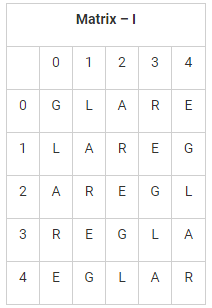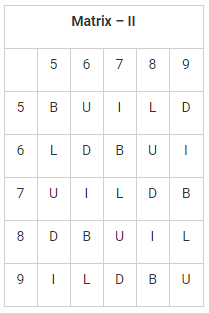Detailed Solution for Test: General Intelligence & Reasoning- 2 - Question 50

1) 00, 68, 95, 58, 04 → GUILE

2) 14, 75, 88, 87, 40 → GUIUE

3) 23, 99, 76, 78, 31 → GUIDE

4) 41, 87, 57, 66, 12 → GUIDR

Hence, “23, 99, 76, 78, 31”is the correct set.

## Mock Test Series of SSC JE Civil Engineering

1 videos|1 docs|64 tests
 Use Code STAYHOME200 and get INR 200 additional OFF Use Coupon Code
Information about Test: General Intelligence & Reasoning- 2 Page
In this test you can find the Exam questions for Test: General Intelligence & Reasoning- 2 solved & explained in the simplest way possible. Besides giving Questions and answers for Test: General Intelligence & Reasoning- 2, EduRev gives you an ample number of Online tests for practice

## Mock Test Series of SSC JE Civil Engineering

1 videos|1 docs|64 tests